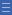# Unequal areas

Unequal areas

An example of this misconception — number of parts only — is when students do not attend to the equality of parts in area diagrams.

When asked to choose the shapes that show one-third, a student with the 'number of parts only' misconception has responded by selecting all the shapes that have three parts.

Typically, students begin their exploration of fractions through using the area model. They are presented with shapes to represent one whole. Students are meant to understand that the area of these shapes can be divided into equal parts to represent fractions.

Difficulties can arise from the early use of the area model for fractions.

• Some students will not yet comprehend the concept of area so will be unsure what is actually being divided into parts.
• Many students will not be ready to compare areas that are different shapes or in different orientations because they have not yet developed the cognitive skill of 'conservation' of area.

The exploration of one-half is often combined with an introduction to line symmetry. While this might assist in understanding symmetry, it might not be as helpful for understanding the concept of half. It is not always the case that the line dividing an area in half will also be a line of symmetry, as in the shapes below.

Yes

Yes

Name Class SectionYear 1: Recognise and describe one-half as one of two equal parts of a whole Infobox 3Year 2: Recognise and interpret common uses of halves, quarters and eighths of shapes and collections Infobox 3